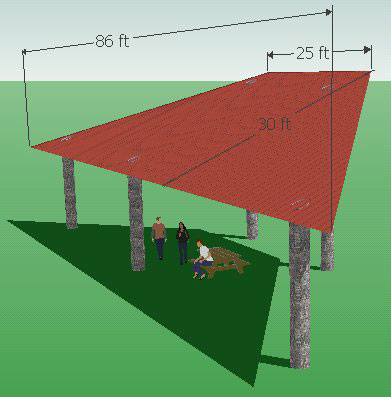SEARCH HOMEMath Central Quandaries & QueriesQuestion from RANDY, a parent: I have an area of roof. It's in a sort of triangle. it's 25' across the top 30' at each edge 86' across the bottomHi Randy. I tried to consider a roof where the edges were 30', but if
the bottom is 86' long, I think the 30' at each edge is the
measurement perpendicular to the top and bottom edges.

Here's a diagram of what I mean:This shows a trapezoidal roof. The area of a trapezoid is the average of the top and bottom lengths times the height.

So your roof is (86 + 25) / 2 x 30 = 915 square feet.

If we have misinterpreted your description let us know.

Cheers,
Stephen La Rocque.Math Central is supported by the University of Regina and The Pacific Institute for the Mathematical Sciences.